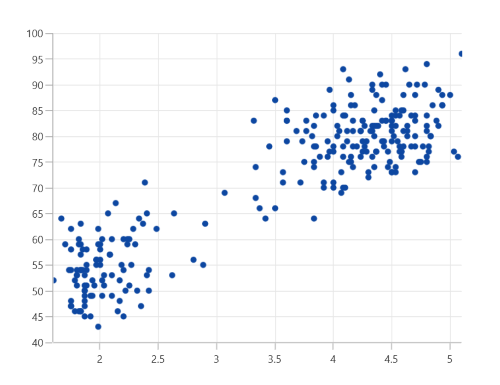# Scatter Chart in WinUI Charts (SfCartesianChart)

15 Sep 20222 minutes to read

The WinUI Scatter Chart is similar to bubble chart, where each data point being represented by a circle with equal size.

## Scatter Chart

To render a scatter chart, create an instance of the ScatterSeries, and add it to the Series collection property of SfCartesianChart. The segment size can be defined by using the PointHeight and PointWidth properties.

``````<chart:SfCartesianChart>

<chart:SfCartesianChart.XAxes>
<chart:NumericalAxis />
</chart:SfCartesianChart.XAxes>

<chart:SfCartesianChart.YAxes>
<chart:NumericalAxis />
</chart:SfCartesianChart.YAxes>

<chart:SfCartesianChart.Series>
<chart:ScatterSeries PointHeight="7"
PointWidth="7"
ItemsSource="{Binding Data}"
XBindingPath="XValue"
YBindingPath="YValue"/>
</chart:SfCartesianChart.Series>

</chart:SfCartesianChart>``````
``````SfCartesianChart chart = new SfCartesianChart();
NumericalAxis xAxis = new NumericalAxis();
NumericalAxis yAxis = new NumericalAxis();

ScatterSeries series = new ScatterSeries()
{
ItemsSource = new ViewModel().Data,
XBindingPath = "XValue",
YBindingPath = "YValue",
PointHeight = 7,
PointWidth = 7,
};